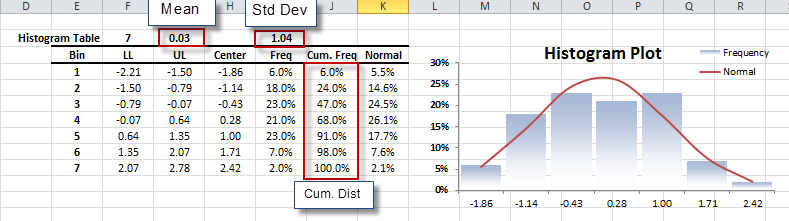# Histogram Analysis

NumXL provides an intuitive interface to help Excel users streamline the decisions involved in constructing a good histogram plot. In this tutorial, we’ll demonstrate the steps to construct a histogram using NumXL functions in Excel.

## Process

1. Select an empty cell to store the histogram table.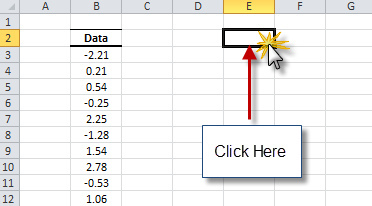2. Locate the Descriptive Statistics (DESC STAT) icon in the toolbar (or menu in Excel 2003) and click on the down-arrow. When the drop-down menu appears, select “Histogram Plot”.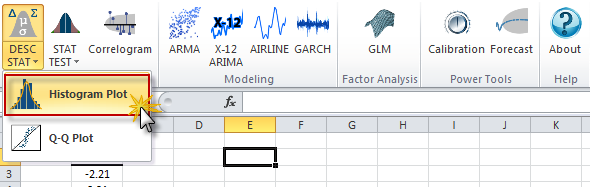3. The Histogram dialog box appears.
4. Select the cell range for the input data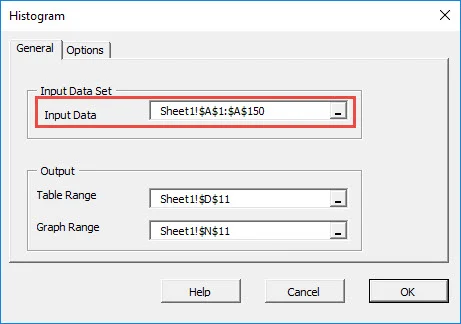Note: The output range (i.e. table) is set by default to the selected empty cell in your worksheet

5. Switch to the Options tab and select the rule/heuristic to determine the optimal number of histogram bins. For our example, we selected “Scott’s Choice”.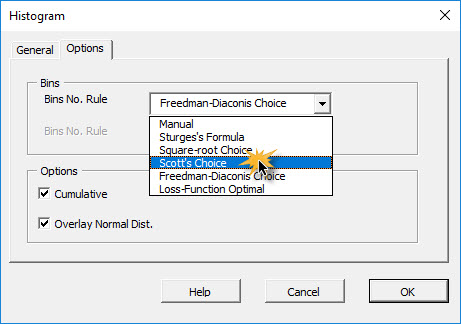6. Immediately below it, the estimated number of bins is shown.
7. Under options, (i) checking “Cumulative” instructs the histogram Wizard to include the cumulative distribution in the output table, while (ii) checking “Overlay Normal” instructs the Wizard to plot a reference Gaussian distribution in the graph.
8. When you finish, click “OK”.

## Output

The wizard generates the histogram frequency table and plots a column-type graph as shown below